# JP6079388B2 - Reception circuit and control method thereof - Google Patents

## Info

Publication number
JP6079388B2
JP6079388B2 JP2013077887A JP2013077887A JP6079388B2 JP 6079388 B2 JP6079388 B2 JP 6079388B2 JP 2013077887 A JP2013077887 A JP 2013077887A JP 2013077887 A JP2013077887 A JP 2013077887A JP 6079388 B2 JP6079388 B2 JP 6079388B2
Authority
JP
Japan
Prior art keywords
circuit
phase
data
equalization
clock signal
Prior art date
Legal status (The legal status is an assumption and is not a legal conclusion. Google has not performed a legal analysis and makes no representation as to the accuracy of the status listed.)
Active
Application number
JP2013077887A
Other languages
Japanese (ja)
Other versions
JP2014204234A (en
Inventor

Original Assignee

Priority date (The priority date is an assumption and is not a legal conclusion. Google has not performed a legal analysis and makes no representation as to the accuracy of the date listed.)
Filing date
Publication date
Application filed by 富士通株式会社 filed Critical 富士通株式会社
Priority to JP2013077887A priority Critical patent/JP6079388B2/en
Publication of JP2014204234A publication Critical patent/JP2014204234A/en
Application granted granted Critical
Publication of JP6079388B2 publication Critical patent/JP6079388B2/en
Application status is Active legal-status Critical
Anticipated expiration legal-status Critical

## Images

•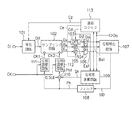•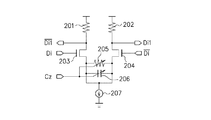••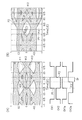•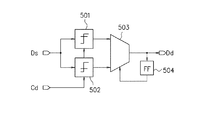••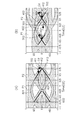•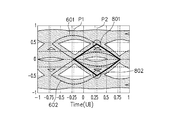•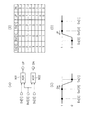•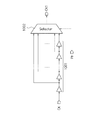•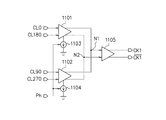•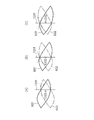••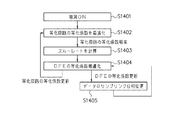## Classifications

• HELECTRICITY
• H03BASIC ELECTRONIC CIRCUITRY
• H03LAUTOMATIC CONTROL, STARTING, SYNCHRONISATION, OR STABILISATION OF GENERATORS OF ELECTRONIC OSCILLATIONS OR PULSES
• H03L7/00Automatic control of frequency or phase; Synchronisation
• H03L7/06Automatic control of frequency or phase; Synchronisation using a reference signal applied to a frequency- or phase-locked loop
• H03L7/08Details of the phase-locked loop
• H03L7/081Details of the phase-locked loop provided with an additional controlled phase shifter
• H03L7/0812Details of the phase-locked loop provided with an additional controlled phase shifter and where no voltage or current controlled oscillator is used
• HELECTRICITY
• H04ELECTRIC COMMUNICATION TECHNIQUE
• H04LTRANSMISSION OF DIGITAL INFORMATION, e.g. TELEGRAPHIC COMMUNICATION
• H04L25/00Baseband systems
• H04L25/02Details ; Arrangements for supplying electrical power along data transmission lines
• H04L25/03Shaping networks in transmitter or receiver, e.g. adaptive shaping networks ; Receiver end arrangements for processing baseband signals
• H04L25/03006Arrangements for removing intersymbol interference
• H04L25/03012Arrangements for removing intersymbol interference operating in the time domain
• H04L25/03019Arrangements for removing intersymbol interference operating in the time domain adaptive, i.e. capable of adjustment during data reception
• H04L25/03057Arrangements for removing intersymbol interference operating in the time domain adaptive, i.e. capable of adjustment during data reception with a recursive structure
• HELECTRICITY
• H04ELECTRIC COMMUNICATION TECHNIQUE
• H04LTRANSMISSION OF DIGITAL INFORMATION, e.g. TELEGRAPHIC COMMUNICATION
• H04L7/00Arrangements for synchronising receiver with transmitter
• H04L7/02Speed or phase control by the received code signals, the signals containing no special synchronisation information
• H04L7/033Speed or phase control by the received code signals, the signals containing no special synchronisation information using the transitions of the received signal to control the phase of the synchronising-signal-generating means, e.g. using a phase-locked loop
• H04L7/0337Selecting between two or more discretely delayed clocks or selecting between two or more discretely delayed received code signals
• HELECTRICITY
• H03BASIC ELECTRONIC CIRCUITRY
• H03LAUTOMATIC CONTROL, STARTING, SYNCHRONISATION, OR STABILISATION OF GENERATORS OF ELECTRONIC OSCILLATIONS OR PULSES
• H03L7/00Automatic control of frequency or phase; Synchronisation
• H03L7/06Automatic control of frequency or phase; Synchronisation using a reference signal applied to a frequency- or phase-locked loop
• H03L7/08Details of the phase-locked loop
• H03L7/085Details of the phase-locked loop concerning mainly the frequency- or phase-detection arrangement including the filtering or amplification of its output signal
• H03L7/091Details of the phase-locked loop concerning mainly the frequency- or phase-detection arrangement including the filtering or amplification of its output signal the phase or frequency detector using a sampling device
• HELECTRICITY
• H04ELECTRIC COMMUNICATION TECHNIQUE
• H04LTRANSMISSION OF DIGITAL INFORMATION, e.g. TELEGRAPHIC COMMUNICATION
• H04L25/00Baseband systems
• H04L25/02Details ; Arrangements for supplying electrical power along data transmission lines
• H04L25/03Shaping networks in transmitter or receiver, e.g. adaptive shaping networks ; Receiver end arrangements for processing baseband signals
• H04L25/03006Arrangements for removing intersymbol interference
• H04L25/03012Arrangements for removing intersymbol interference operating in the time domain
• H04L25/03019Arrangements for removing intersymbol interference operating in the time domain adaptive, i.e. capable of adjustment during data reception

## Description

The present invention relates to a receiving circuit and a control method thereof.

As the performance of information processing devices such as communication backbone devices and servers increases, it is necessary to increase the data rate of signal transmission and reception inside and outside the device. The reception circuit recovers a clock signal and data from data having a high data rate (CDR: Clock and Data Recovery).

A decision feedback type equalizer having an odd data receiver and an even data receiver is known (see, for example, Patent Document 1). The odd data receiving unit has a half-rate DFE equalization function that receives an odd data sampling clock, an odd edge sampling clock, and a DFE input signal. The even data receiving unit has a half rate DFE equalization function that receives an even data sampling clock, an even edge sampling clock, and a DFE input signal. Each of the odd data reception unit and the even data reception unit has a data detection means and an edge detection means with a half-rate DFE equalization signal, and receives a sampling data group by the detection means as a continuous 3-bit data detection A data pattern of 110 or 001 is detected. The pattern filter selects edge data in the half-rate DFE equalization signal only when a 110 or 001 pattern is detected based on the detection result.

A receiving circuit having a sampling circuit that samples an input data signal based on a clock signal and outputs a sampling signal is known (for example, see Patent Document 2). The data interpolation circuit interpolates the sampling signal based on the phase information of the sampling signal with respect to the input data signal and outputs an interpolation data signal. The interpolation error determination circuit outputs an interpolation error based on the sampling signal and the phase information. The determination equalization circuit equalizes the interpolation data signal with an equalization coefficient set based on the interpolation error, determines the equalized interpolation data signal, and outputs a determination signal. The phase detection circuit generates phase information based on the determination signal or the equalized interpolation data signal, and outputs the phase information to the data interpolation circuit and the interpolation error determination circuit.

JP 2009-22018 A JP 2012-124593 A

In the equalization circuit, a phase shift occurs according to the equalization amount. In a system that uses a decision feedback equalization circuit that performs equalization after sampling, this phase shift causes a shift from the optimum sampling phase, so that the decision error rate increases.

An object of the present invention is to provide a receiving circuit capable of reducing a determination error rate by sampling data at an appropriate phase and making a determination, and a control method therefor.

The receiving circuit samples the boundary data in synchronization with the first clock signal and samples the center data in synchronization with the second clock signal with respect to the input data signal, and an equalization coefficient. A decision feedback equalization circuit for equalizing and binary-determining the center data sampled by the sampling circuit, a first comparison circuit for binary-determining boundary data sampled by the sampling circuit, the decision feedback, etc. A phase detection circuit for detecting phase information of the input data signal based on output data of the equalization circuit and the first comparison circuit, and a decision feedback equalization circuit based on an equalization coefficient of the decision feedback equalization circuit A phase difference calculation circuit for calculating a phase difference of output data, and the first clock based on the phase information detected by the phase detection circuit; The phase of the second clock signal is adjusted based on the first phase adjustment circuit for adjusting the phase of the signal, the phase information detected by the phase detection circuit, and the phase difference calculated by the phase difference calculation circuit And a second phase adjustment circuit.

By adjusting the phase of the second clock signal based on the phase difference calculated by the phase difference calculation circuit, it is possible to determine the center data of an appropriate phase and reduce the determination error rate. it can.

FIG. 1 is a diagram illustrating a configuration example of a receiving circuit according to the present embodiment. FIG. 2 is a circuit diagram showing a configuration example of the equalization circuit of FIG. FIG. 3 is a circuit diagram showing a configuration example of the sampling circuit of FIG. 4A shows the output of the sampling circuit, and FIG. 4B shows the output of the decision feedback equalization circuit. FIG. 5 is a diagram illustrating a configuration example of the decision feedback equalization circuit of FIG. 6A is a diagram showing the output of the sampling circuit, and FIG. 6B is a diagram showing the output of the decision feedback equalization circuit. FIG. 7A is a diagram showing the output of the sampling circuit, and FIG. 7B is a diagram showing the output of the decision feedback equalization circuit. FIG. 8 is a diagram for explaining a calculation method of the phase difference calculation circuit of FIG. 9A to 9D are diagrams for explaining a configuration example of the phase detection circuit of FIG. FIG. 10 is a circuit diagram showing a configuration example of the first phase adjustment circuit of FIG. FIG. 11 is a circuit diagram showing another configuration example of the first phase adjustment circuit of FIG. 12A to 12C are diagrams for explaining the convergence of the receiving circuit in FIG. 13A to 13F are diagrams illustrating simulation results of convergence of the receiving circuit in FIG. FIG. 14 is a flowchart showing a control method of the receiving circuit of FIG.

FIG. 1 is a diagram illustrating a configuration example of a receiving circuit according to the present embodiment. The reception circuit is, for example, a reception circuit of a high-speed input / output I / O circuit that transmits and receives a signal with a high bit rate within an integrated circuit chip or between chips (inside or between devices). The receiving circuit reproduces a clock signal and data (CDR: Clock and Data Recovery) based on the input data signal Di having a high data rate.

The equalization circuit 101 equalizes the input data signal Di based on the equalization coefficient Cz, and outputs the equalized input data signal Di1 to the sampling circuit 102. The receiving circuit inputs the input data signal Di from the transmitting circuit via the transmission path. When the transmission circuit transmits a binary pulse signal, the reception circuit inputs an input data signal Di having a slow rising speed and falling speed of the pulse signal due to the characteristics of the transmission path. The equalization circuit 101 equalizes the input data signal Di so as to remove the characteristics of the transmission path, thereby outputting the input data signal Di1 that is close to the transmission pulse signal of the transmission circuit. The input data signal Di1 is a signal having a faster rising speed and / or falling speed than the input data signal Di.

FIG. 2 is a circuit diagram showing a configuration example of the equalization circuit 101 of FIG. Although FIG. 1 shows an example of a single-ended signal, the equalizer circuit 101 of FIG. 2 shows an example of a differential signal. The differential input data signals Di and / Di are signals whose phases are inverted from each other. The differential input data signals Di1 and / Di1 are signals whose phases are inverted from each other. The equalization circuit 101 receives the differential input data signals Di and / Di and outputs the differential input data signals Di1 and / Di1.

Resistor 201 is connected between the power supply voltage node and the node of input data signal / Di1. The resistor 202 is connected between the power supply voltage node and the node of the input data signal Di1. N-channel field effect transistor 203 has a drain connected to the node of input data signal / Di1, and a gate connected to a node of input data signal Di. N-channel field effect transistor 204 has a drain connected to a node of input data signal Di1, and a gate connected to a node of input data signal / Di. The variable resistor 205 is connected between the source of the transistor 203 and the source of the transistor 204, and the resistance value changes according to the equalization coefficient Cz. The variable capacitor 206 is connected between the source of the transistor 203 and the source of the transistor 204, and the capacitance value changes according to the equalization coefficient Cz. Constant current source 207 is connected between the interconnection point of the sources of transistors 203 and 204 and the ground potential node.

In FIG. 2, a CTLE (Continuous Time Linear Equalizer) circuit is shown as an example of the equalization circuit 101. In this circuit, the variable resistor 205 between the sources of the differential input transistors 203 and 204 lowers the gain of the low frequency component, enhances the high frequency component equivalently, and restores the high frequency component of the input data signal. The emphasized gain and frequency characteristics are adjusted by changing the capacitance value of the variable capacitor 206 and / or the resistance value of the variable resistor 205 according to the equalization coefficient Cz.

The sampling circuit 102 in FIG. 1 samples the boundary data Bs in synchronization with the first clock signal CK1 with respect to the input data signal Di1, and the center data Ds and error data in synchronization with the second clock signal CK2. Sampling Es.

FIG. 3 is a circuit diagram showing a configuration example of the sampling circuit 102 of FIG. The switch 302 connects the node of the input data signal Di1 to the capacitor 304 when the first clock signal CK1 becomes high level, and connects the node of the input data signal Di1 to the capacitor 304 when the first clock signal CK1 becomes low level. Disconnect from. The switch 301 connects the node of the input data signal Di1 to the capacitor 303 when the second clock signal CK2 becomes high level, and connects the node of the input data signal Di1 to the capacitor 303 when the second clock signal CK2 becomes low level. Disconnect from. The operational amplifier 306 amplifies the voltage of the capacitor 304 and outputs the boundary data Bs. The operational amplifier 305 amplifies the voltage of the capacitor 303 and outputs center data Ds and error data Es. The center data Ds and the error data Es are the same data.

The clock signals CK1 and CK2 are signals whose phases are reversed. The sampling circuit 102 interleaves with the clock signals CK1 and CK2. As shown in FIG. 4A, the sampling circuit 102 outputs the boundary data 401 (Bs) by sampling the input data signal Di1 at the timing P1 in synchronization with the rising edge of the clock signal CK1. In synchronization with the rising edge of CK2, the center data Ds is output by sampling the input data signal Di1 at timing P2. Note that the number of interleaves is not limited to two, and can be 2 × n (n is an integer), and is determined according to the operation speed of the circuit. 4A shows an example of the two-phase clock signals CK1 and CK2, a four-phase clock signal whose frequency is halved may be used.

4A, in the waveform of the input data signal Di1, the horizontal axis represents time, and the vertical axis represents the amplitude value. The time on the horizontal axis is indicated by a unit interval (UI). 1 UI is a 1-bit time of the input data signal Di1, and is a time between two adjacent data boundary times (data transition times). The data having a period of 1 [UI] indicates a data transition state of “101010. Data with a period of 2 [UI] indicates a data transition state of “11001100. Timing P1 is a boundary data timing indicating the timing of the boundary between two adjacent data. Timing P2 is center data timing indicating the timing of the center of one piece of data. The timings P1 and P2 have a phase difference of 0.5 [UI] from each other.

The decision feedback equalizer (DFE: Decision Feedback Equalizer) 103 in FIG. 1 equalizes and binary-determines the center data Ds sampled by the sampling circuit 102 using the equalization coefficient Cd to obtain binary center data. Dd is output. The center data Dd is a binary value of “0” or “1”. For example, data “1” is data with a positive amplitude value, and data “0” is data with a negative amplitude value. The equalization circuit 101 is a primary equalization circuit, and the decision feedback equalization circuit 103 is a secondary equalization circuit.

FIG. 5 is a diagram illustrating a configuration example of the decision feedback equalization circuit 103 in FIG. The decision feedback equalization circuit 103 receives the equalization coefficient Cd, gives the first equalization coefficient “+ Cd” to the comparison circuit 501, and gives the second equalization coefficient “−Cd” to the comparison circuit 502. The comparison circuit 501 outputs a comparison result between the center data Ds and the first equalization coefficient “+ Cd”. Specifically, the comparison circuit 501 outputs data “1” when the center data Ds is larger than the first equalization coefficient “+ Cd”, and the center data Ds is obtained from the first equalization coefficient “+ Cd]. If it is smaller, data “0” is output. The comparison circuit 502 outputs a comparison result between the center data Ds and the second equalization coefficient “−Cd”. Specifically, the comparison circuit 502 outputs data “1” when the center data Ds is larger than the second equalization coefficient “−Cd”, and the center data Ds becomes the second equalization coefficient “−Cd”. ], Data “0” is output. The selector 503 selects the output data of the comparison circuit 501 when the data stored in the flip-flop circuit 504 is “1” and the data stored in the flip-flop circuit 504 is “0”. Selects the output data of the comparison circuit 502 and outputs binary data Dd. The flip-flop circuit 504 stores the output data Dd of the selector 503. That is, the selector 503 selects the output data of the comparison circuit 501 or 502 according to the previous output data Dd stored in the flip-flop circuit 504.

FIG. 6A is a diagram corresponding to FIG. The data transition 601 indicates a transition from the previous data “1” to the current data “1” or “0” in the data pattern “11001100...”. In this case, the center amplitude value 411 of the data transition 601 is located above the amplitude value “0”. Therefore, when the previous data is “1”, the comparison circuit 501 outputs the data “1” when it is larger than the first equalization coefficient 411 (= “+ Cd”), and the first equalization. If the coefficient is smaller than 411 (= “+ Cd”), data “0” is output.

The data transition 602 indicates a transition from the previous data “0” to the current data “1” or “0” in the data pattern “11001100...”. In this case, the center amplitude value 413 of the data transition 602 is located below the amplitude value “0”. Therefore, when the previous data is “0”, the comparison circuit 502 outputs data “1” when the second equalization coefficient 413 (= “− Cd”) is larger than the second equalization coefficient 413 (= “− Cd”). If it is smaller than the conversion factor 413 (= “− Cd”), data “0” is output.

The decision feedback equalization circuit 103 in FIG. 5 is a one-tap decision feedback equalization circuit. The selector 503 can perform high-speed processing by selecting according to the previous output data Dd. The decision feedback equalization circuit 103 is not limited to one tap, and can be realized with a plurality of taps. For example, in the case of the 2-tap decision feedback equalization circuit 103, four comparison circuits 501, 502 are provided, and the four comparison circuits 501, 502 are provided according to the output data Dd one bit before and two bits before. Etc., one of the output data is selected. Similarly, in the case of the m-tap decision feedback equalization circuit 103, 2 m comparison circuits 501 and 502 are required.

6A is a diagram illustrating output data of the sampling circuit 102, and FIG. 6B is a diagram illustrating output data of the decision feedback equalization circuit 103. FIG. As shown in FIG. 6B, the determination feedback equalization circuit 103 is equivalent to determining the data of the data transitions 601 and 602 using the threshold value 412 of the amplitude value “0”. Data 401 at timing P1 in FIG. 6A is boundary data Bs. The data 403 at the timing P2 in FIG. 6B is the center data Dd. The center data timing P2 in FIG. 6A is located at the center phase of the data, but the data 403 at the center data timing P2 in FIG. 6B is at a position (right side position) delayed from the data center phase. It's off. This is due to the equalization processing of the decision feedback equalization circuit 103. The determination feedback equalization circuit 103 decreases the determination error rate if the sampling data of the center phase of the data is determined, but increases the determination error rate if the sampling data 403 at the shifted timing P2 is determined. A method for reducing the determination error rate of the determination feedback equalization circuit 103 by adjusting the sampling timing P2 of the center data 403 will be described below.

The first comparison circuit 104 in FIG. 1 performs binary determination on the boundary data Bs sampled by the sampling circuit 102 and outputs binary boundary data. Specifically, the first comparison circuit 104 outputs “1” when the amplitude value of the boundary data Bs is larger than “0”, and outputs the value when the amplitude value of the boundary data Bs is smaller than “0”. “0” is output.

The second comparison circuit 105 compares the error data Es (= center data Ds) sampled by the sampling circuit 102 with the threshold value Ce, and outputs binary error data. Specifically, the second comparison circuit 105 outputs error data “1” when the absolute value of the error data Es is smaller than the threshold value Ce, and the absolute value of the error data Es is larger than the threshold value Ce. In this case, error data “0” is output.

The demultiplexing circuit 106 demultiplexes the output data of the decision feedback equalization circuit 103, the first comparison circuit 104, and the second comparison circuit 105, and outputs center data Do, boundary data Bs1, and error data Es1, respectively. To do. That is, the demultiplexing circuit 106 converts a high frequency serial signal into a low frequency parallel signal. Since the output data of the demultiplexing circuit 106 has a low data rate, the subsequent phase detection circuit 107, phase difference calculation circuit 109, and adaptive logic circuit 113 can perform low-speed processing. The center data Do is output data of the receiving circuit.

The adaptive logic circuit 113 in FIG. 1 calculates the equalization coefficient Cz, the threshold value Ce, and the equalization coefficient Cd based on the center data Do and the error data Es1, and outputs the equalization coefficient Cz, the threshold value Ce, and the equalization coefficient Cd. To do. The adaptive logic circuit 113 optimizes the equalization coefficient Cd and the like sequentially using, for example, an LMS (Least Mean Square) algorithm. For example, the adaptive logic circuit 113 calculates the equalization coefficient Cd (n−1) of the (n−1) th sampling data, the error data Es (n) of the nth sampling data, and the (n−1) th center by the following equation. Using the data Do (n−1), the equalization coefficient Cd (n) of the nth sampling data can be derived. Here, μ represents a step. The adaptive logic circuit 113 calculates the equalization coefficient Cz in the same manner as the equalization coefficient Cd.
Cd (n) = Cd (n−1) −μ × Es1 (n) × Do (n−1)

FIG. 7A corresponds to FIG. 6A and shows the output of the sampling circuit 102. The boundary data 401 is located at the phase of the zero cross point where the data transitions 601 and 602 cross at the amplitude value “0”. The solid line portions of the data transitions 601 and 602 near the zero cross point can be approximated to a straight line. Here, the first equalization coefficient 411 is an equalization coefficient Cd, which is a known value. Here, the slope of the straight line portion of the data transitions 601 and 602 is a slew rate (waveform slope). Therefore, if the slope of the straight line is known, the phase difference 702 can be calculated based on the slope of the straight line and the equalization coefficient Cd.

FIG. 7B corresponds to FIG. 6B and shows the output of the decision feedback equalization circuit 103. The center data 403 is equalized by the decision feedback equalization circuit 103 as described above. Thus, there is a phase difference 702 shift with respect to the center phase of the data. As described above, the phase difference 702 can be calculated based on the slope of the straight line and the equalization coefficient Cd. By advancing the phase of the center data 403 by the phase difference 702, the center data 402 of the data center phase can be obtained. The determination feedback equalization circuit 103 can reduce the determination error rate by determining the center data 402 of the data center phase.

The phase difference calculation circuit 109 in FIG. 1 calculates the phase difference 702 described above. Specifically, the phase difference calculation circuit 109 calculates the phase difference Sh of the output data of the decision feedback equalization circuit 103 based on the equalization coefficient Cd of the decision feedback equalization circuit 103, the error data Es1, and the center data Do. Calculate. The phase difference Sh corresponds to the phase difference 702 in FIG.

FIG. 8 corresponds to FIG. 7A and is a diagram for explaining a calculation method of the phase difference calculation circuit 109 in FIG. The phase difference calculation circuit 109 detects linear approximate cross points 801 and 802 of the data transitions 601 and 602 at the sampling timing P2. After 0.5 [UI] of the timing P2, the data transitions 601 and 602 cross with an amplitude value “0”. The solid line portion of the data transitions 601 and 602 that passes through the above cross points 801 and 802 can be approximated to a straight line. The adaptive logic circuit 113 changes the threshold value Ce, the second comparison circuit 105 compares the absolute value of the error data Es (center data Ds) with the threshold value Ce, and the phase difference calculation circuit 109 is the second comparison circuit. The comparison result 105 is input. As a result, the phase difference calculation circuit 109 can know whether or not the center data Ds at the timing P2 is within the range of + Cs to −Cs. By changing the threshold value Cs, the phase difference calculation circuit 109 can know the amplitude values of the cross points 801 and 802. The threshold value Cs can be used together with the error monitoring threshold value of the adaptive logic circuit 113.

For example, the amplitude value of the cross point 801 is detected as “0.42.” The phase difference calculation circuit 109 inputs “0.22” as the equalization coefficient Cd from the adaptive logic circuit 113. In this case, the slew rate (slope) of the solid line connecting the cross point 801 and the zero cross point after 0.5 [UI] is set to 0.42 / 0.5 = 0.84 by the phase difference calculation circuit 109. Calculated. Next, the phase difference calculation circuit 109 calculates the phase difference 702 (Sh) by the calculation of 0.22 / 0.84 = 0.26.

Specifically, the phase difference calculation circuit 109 derives the phase difference Sh by performing the following calculation, for example. Here, Cd is an equalization coefficient, and AC is an absolute value of the amplitude value of the cross point 801 or 802.
Sh = Cd / (2 × AC)

The phase detection circuit 107 in FIG. 1 receives the center data Do and the boundary data Bs1, and detects and outputs the phase information UD of the input data signal Di based on the center data Do and the boundary data Bs1.

FIG. 9A is a circuit diagram illustrating a configuration example of the phase detection circuit 107 of FIG. The phase detection circuit 107 includes exclusive OR (XOR) circuits 901 and 902. As shown in FIGS. 9C and 9D, the center data Do  is the center data Do of the first cycle. The center data Do  is the center data Do of the next second cycle. The boundary data Bs1  is boundary data Bs1 between the center data Do  and Do . The exclusive OR circuit 901 outputs the exclusive OR data of the center data Do  and the boundary data Bs1  as the bit UP. The exclusive OR circuit 902 outputs the exclusive OR data of the center data Do  and the boundary data Bs1  as the bit DN. As shown in FIG. 9B, the phase detection circuit 107 receives data Do , Do , and Bs1  and outputs 2-bit phase data UP and DN. The 2-bit phase data UP and DN correspond to the phase information UD in FIG.

FIG. 9C is a diagram illustrating an example in which the phase of the down-boundary data Bs1  is earlier than the boundary phase (data transition phase). For example, the center data Do  and the boundary data Bs1  are “1”, and the next center data Do  is “0”. In this case, since the phase of the down boundary data Bs1  is earlier than the boundary phase (data transition phase), the down bit DN of “1” is output to delay the sampling phase.

FIG. 9D is a diagram illustrating an example in which the phase of the down data Bs1  is later than the boundary phase (data transition phase). For example, the center data Do  is “0”, the boundary data Bs1  and the next center data Do  are “1”. In this case, since the phase of the down data Bs1  is later than the boundary phase (data transition phase), the up bit UP of “1” is output to advance the sampling phase.

As described above, the phase detection circuit 107 determines whether the boundary data Bs1  is the same as the previous center data Do  or the subsequent center data Do  when the data value changes. To detect a phase shift. In FIGS. 9A to 9D, only the configuration between continuous 2-bit data is shown. Actually, however, the demultiplexing circuit 106 converts the data into a plurality of bits. Perform phase detection.

A filter 108 in FIG. 1 is a low-pass filter, and low-pass filters the phase information UD detected by the phase detection circuit 107 and outputs phase information Ph. The filter 108 can remove the high frequency noise and output the averaged phase information Ph. By using the filter 108, a sudden change in the phase information Ph can be prevented, and the change in the phase information Ph can be moderated.

The first phase adjustment circuit 111 adjusts the phase of the first clock signal CK1 using the reference clock signal CK according to the phase information Ph. When the up bit UP is “1”, control is performed so that the phase of the first clock signal CK1 is advanced. When the down bit DN is “1”, the phase of the first clock signal CK1 is controlled to be delayed. Thus, the phase of the boundary data Bs1  in FIGS. 9C and 9D is controlled so as to coincide with the boundary phase (data transition phase).

The subtracter 110 adds the phase information Ph and 0.5 [UI], and outputs the phase information Ph1 by subtracting the phase difference Sh from the addition result. The second phase adjustment circuit 112 adjusts the phase of the second clock signal CK2 using the reference clock signal CK according to the phase information Ph1. Similarly to the above, when the up bit UP is “1”, the phase of the second clock signal CK2 is controlled to be advanced. When the down bit DN is “1”, the phase of the second clock signal CK2 is controlled to be delayed. As shown in FIG. 4A, since the center data phase P2 is a phase delayed by 0.5 [UI] with respect to the boundary data phase P1, the subtractor 110 adds 0.5 [UI]. To do. Further, the phase difference Sh corresponds to the phase difference 702 in FIG. As shown in FIG. 7B, the subtractor 110 can change the phase of the center data 403 to an appropriate phase of the center data 402 by subtracting the phase difference 702 (Sh).

In FIG. 4A, the first clock signal CK1 and the second clock signal CK2 are examples of clock signals before phase adjustment, and the first clock signal CK1a and the second clock signal CK2a are after phase adjustment. An example of a clock signal is shown. Before the phase adjustment, the second clock signal CK2 is delayed by 0.5 [UI] with respect to the first clock signal CK1. Here, when the phase difference Sh is calculated by the phase difference calculation circuit 109, the phase of the first clock signal CK1a after the phase adjustment is the same as that of the first clock signal CK1 before the phase adjustment. On the other hand, the second clock signal CK2a after the phase adjustment is adjusted to a phase advanced by the phase difference Sh with respect to the second clock signal CK2 before the phase adjustment. After the phase adjustment, the sampling circuit 102 samples the boundary data Bs in synchronization with the rising phase P1 of the first clock signal CK1a, and the center data Ds and the error data in synchronization with the rising phase P3 of the second clock signal CK2a. Data Es is sampled.

FIG. 4B corresponds to FIG. 7B and shows an output example of the decision feedback equalization circuit 103 when sampling is performed using the first clock signal CK1a and the second clock signal CK2a after phase adjustment. It is. The determination feedback equalization circuit 103 determines the center data 402 sampled at the phase P3. Since the center data 402 is adjusted to the center phase P3, the determination error rate of the determination feedback equalization circuit 103 can be reduced.

FIG. 10 is a circuit diagram showing a configuration example of the first phase adjustment circuit 111 of FIG. The first phase adjustment circuit 111 is configured by a variable delay circuit. A series connection circuit 1001 of a plurality of buffers is a delay circuit, and delays the reference clock signal CK. The selector 1002 selects any one of the plurality of buffers 1001 according to the phase information Ph and outputs the first clock signal CK1. The first clock signal CK1 is a signal obtained by delaying the reference clock signal CK by a delay time corresponding to the phase information Ph.

FIG. 11 is a circuit diagram showing another configuration example of the first phase adjustment circuit 111 of FIG. The first phase adjustment circuit 111 is configured by a phase interpolation circuit. The four-phase reference clock signals CK0, CL90, CL180, and CL270 correspond to the reference clock signal CK in FIG. The clock signal CK0 is a clock signal having a phase of 0 degrees, the clock signal CL90 is a clock signal having a phase of 90 degrees, the clock signal CL180 is a clock signal having a phase of 180 degrees, and the clock signal CL270 is a phase of 270 degrees. Clock signal. The differential amplifier 1101 is connected to the current source 1103, amplifies the differential clock signals CL0 and CL180, and outputs the differential clock signals at the nodes N1 and N2. The differential amplifier 1102 is connected to the current source 1104, amplifies the differential clock signals CL90 and CL270, and outputs the differential clock signals of the nodes N1 and N2. The differential amplifier 1105 amplifies the differential clock signals at the nodes N1 and N2, and outputs differential clock signals CK1 and / CK1. The differential clock signals CK1 and / CK1 are signals whose phases are inverted with each other, and correspond to the first clock signal CK1 in FIG.

The current ratios of the current sources 1103 and 1104 are controlled according to the phase information Ph. The output signal of the differential amplifier 1101 and the output signal of the differential amplifier 1102 are added at the nodes N1 and N2 with weighting according to the current ratio.

When the current ratio between the current sources 1103 and 1104 is 1: 0, the first clock signal CK1 has the same phase as the 0-degree clock signal CL0. The first clock signal / CK1 has the same phase as the 180-degree clock signal CL180.

When the current ratio between the current sources 1103 and 1104 is 0: 1, the first clock signal CK1 has the same phase as the 90-degree clock signal CL90. The first clock signal / CK1 has the same phase as the 270-degree clock signal CL270.

As described above, by controlling the current ratio of the current sources 1103 and 1104 according to the phase information Ph, it is possible to generate the first clock signal CK1 or / CK1 having an arbitrary phase of 0 degrees to 360 degrees. it can.

In the above, the configuration of the first phase adjustment circuit 111 has been described as an example. However, the configuration of the second phase adjustment circuit 112 is the same as the configuration of the first phase adjustment circuit 111.

12A to 12C are diagrams for explaining the convergence of the receiving circuit in FIG. 12A shows a case where the equalization coefficient Cd of the decision feedback equalization circuit 103 is small, and FIG. 12B shows a case where the equalization coefficient Cd of the decision feedback equalization circuit 103 is an optimum value. 12 (C) shows a case where the equalization coefficient Cd of the decision feedback equalization circuit 103 is large. The equalization coefficient Cd is adjusted so that the aperture in the amplitude direction of the region surrounded by the data transitions 601 and 602 is maximized in the sampling phase. That is, the equalization coefficient Cd that converges for each sampling phase is different. In other words, it can be said that the convergence phase differs for each equalization coefficient Cd. A phase at which the equalization coefficient Cd converges is indicated as a coefficient convergence phase 1201. Phase 1202 indicates the sampling phase that is calculated and shifted from the current slew rate. When the equalization coefficient Cd in FIG. 12A is small, the rising slew rate of the waveform of the data transition 602 is small, the sampling phase 1202 is to the left of the coefficient convergence phase 1201, and the equalization coefficient Cd is controlled in the up direction. . When the equalization coefficient Cd in FIG. 12C is large, conversely, the falling slew rate of the waveform of the data transition 601 is small, the sampling phase 1202 is to the right of the coefficient convergence phase 1201, and the equalization coefficient Cd is Controlled in the down direction. Finally, as shown in FIG. 12 (B), the rising of the waveform of the data transition 602 and the falling slew rate of the waveform of the data transition 601 converge to the phases 1201 and 1202, so that the operation is stable. . In FIG. 12B, the phase is closed to the phase 1202 and the equalization coefficient Cd where the area of the opening of the region surrounded by the data transitions 601 and 602 is maximized.

FIGS. 13A to 13F correspond to FIGS. 12A to 12C and are graphs showing simulation results of convergence of the receiving circuit of FIG. 1, and equalization coefficients Cd are 0 and 0, respectively. .1, 0.2, 0.3, 0.4, 0.5 are shown. When sampling is performed at the coefficient convergence phase AA, the transition probabilities of up-up and down-DN of the equalization coefficient Cd become equal and converge. When the phase difference calculation circuit 109 is not provided, the convergence is performed with the equalization coefficient Cd in FIG. 13C being 0.2. In this case, as in FIG. 6B, since the coefficient convergence phase AA is delayed from the center phase, the determination error rate becomes high.

In contrast, when the phase difference calculation circuit 109 is provided, the equalization coefficient Cd is increased when the equalization coefficient Cd of FIGS. 13A to 13D is 0.1 to 0.3. When the equalization coefficient in FIG. 13F is 0.5, the equalization coefficient Cd is controlled to down DN. In the sampling phase when the equalization coefficient Cd is small, the equalization coefficient Cd is controlled to UP, and in the sampling phase when the equalization coefficient Cd is large, the equalization coefficient Cd is controlled to down DN. Eventually, the equalization coefficient Cd in FIG. 13E converges to a state of 0.4, and the determination error rate decreases as shown in FIG. 4B.

FIG. 14 is a flowchart showing a control method of the receiving circuit of FIG. In step S1401, the receiving circuit is turned on. Next, in step S1402, the adaptive logic circuit 113 converges the equalization coefficient Cz of the equalization circuit 101 by feedback processing for optimizing the equalization coefficient Cz of the equalization circuit 101. At this time, the adaptive logic circuit 113 can improve the detection accuracy of the phase difference Sh by converging the equalization coefficient so that the linearity is improved in the data monitored for the slew rate calculation of the phase difference arithmetic circuit 109. it can. Note that the equalization of the equalization circuit 101 does not require the equalization accuracy of the data having the period of “1010” in order to further improve the accuracy of the above linear approximation, but the cycles of “0011” and “1100”. It is preferable that the data have equalization accuracy.

After convergence of the equalization coefficient Cz, in step S1403, the phase difference calculation circuit 109 calculates a slew rate. After that, in step S1404, the adaptive logic circuit 113 starts the optimization process of the equalization coefficient Cd of the decision feedback equalization circuit 103, and generates the equalization coefficient Cd.

When the equalization coefficient Cd is updated, in step S1405, the second phase adjustment circuit 112 adjusts the phase of the second clock signal CK2 according to the phase difference Sh calculated by the phase difference calculation circuit 109. The sampling circuit 102 changes the sampling phase of the center data Ds and the error data Es. Thereafter, the process returns to step S1404.

If the adaptive logic circuit 113 updates the equalization coefficient Cz of the equalization circuit 101 in step S1404, the process returns to step S1402.

The control method of this embodiment is not limited to the above control method. For example, the optimization process of the equalization coefficient Cz of the equalization circuit 101 in step S1402 is performed during the initial operation, and thereafter, the equalization coefficient Cz of the equalization circuit 101 is not updated until an error occurs. Good. If an error occurs in the optimization process of the equalization coefficient Cd of the decision feedback equalization circuit 103 in step S1404, the adaptive logic circuit 113 returns to step S1402 to update the equalization coefficient Cz of the equalization circuit 101. I do.

In the initial operation, the equalization circuit 101 preferably inputs an input data signal Di including a specific data pattern “0011” or “1100” as a training sequence. By inputting many “0011” or “1100” data patterns that are advantageous for detecting the slew rate as the input data signal Di, the time required for calibration of the initial operation can be shortened. The input data signal Di preferably includes many specific data patterns in order to improve detection accuracy, but is not limited to this.

According to the present embodiment, the center data Ds having an appropriate phase is determined by adjusting the phase of the second clock signal CK2 based on the phase difference Sh calculated by the phase difference calculation circuit 109. And the determination error rate can be reduced.

The above-described embodiments are merely examples of implementation in carrying out the present invention, and the technical scope of the present invention should not be construed in a limited manner. That is, the present invention can be implemented in various forms without departing from the technical idea or the main features thereof.

101 equalization circuit 102 sampling circuit 103 decision feedback equalization circuit 104 first comparison circuit 105 second comparison circuit 106 demultiplexing circuit 107 phase detection circuit 108 filter 109 phase difference calculation circuit 110 subtractor 111 first phase adjustment Circuit 112 Second phase adjustment circuit 113 Adaptive logic circuit

## Claims (9)

1. A sampling circuit that samples boundary data in synchronization with a first clock signal and samples center data in synchronization with a second clock signal with respect to an input data signal;
A decision feedback equalization circuit that equalizes and binary-determines the center data sampled by the sampling circuit using an equalization coefficient;
A first comparison circuit for binary-determining boundary data sampled by the sampling circuit;
A phase detection circuit for detecting phase information of the input data signal based on output data of the decision feedback equalization circuit and the first comparison circuit;
A phase difference calculation circuit for calculating a phase difference of output data of the determination feedback equalization circuit based on an equalization coefficient of the determination feedback equalization circuit;
A first phase adjustment circuit for adjusting a phase of the first clock signal based on phase information detected by the phase detection circuit;
And a second phase adjustment circuit that adjusts the phase of the second clock signal based on the phase information detected by the phase detection circuit and the phase difference calculated by the phase difference calculation circuit. Receiver circuit.
2. And a second comparison circuit for comparing the center data sampled by the sampling circuit with a threshold value.
The phase difference calculation circuit calculates a phase difference of output data of the decision feedback equalization circuit based on an equalization coefficient of the decision feedback equalization circuit and a comparison result of the second comparison circuit. The receiving circuit according to claim 1.
3. The equalization coefficient has a first equalization coefficient and a second equalization coefficient,
The determination feedback equalization circuit is configured to compare the center data sampled by the sampling circuit with the first equalization coefficient or the center data sampled by the sampling circuit according to the previous output data 3. The receiving circuit according to claim 1, wherein a comparison result with the second equalization coefficient is output.
4.   The receiving circuit according to claim 1, wherein the sampling circuit receives an input data signal including a specific data pattern during an initial operation.
5. Furthermore, it has an equalization circuit for equalizing the input data signal,
The receiving circuit according to claim 1, wherein the sampling circuit inputs an input data signal equalized by the equalizing circuit.
6. Furthermore, it has a filter for filtering the phase information detected by the phase detection circuit,
The first phase adjustment circuit adjusts a phase of the first clock signal based on a phase filtered by the filter;
2. The second phase adjustment circuit adjusts the phase of the second clock signal based on the phase filtered by the filter and the phase difference calculated by the phase difference calculation circuit. The receiving circuit according to any one of?
7.   The adaptive logic circuit according to any one of claims 1 to 6, further comprising an adaptive logic circuit that calculates an equalization coefficient of the decision feedback equalization circuit based on output data of the decision feedback equalization circuit. Receiver circuit.
8. And a demultiplexing circuit that demultiplexes output data of the decision feedback equalization circuit and the first comparison circuit,
The receiving circuit according to claim 1, wherein the phase detection circuit detects phase information of the input data signal based on output data of the demultiplexing circuit.
9. A sampling circuit that samples boundary data in synchronization with a first clock signal and samples center data in synchronization with a second clock signal with respect to an input data signal;
A decision feedback equalization circuit that equalizes and binary-determines the center data sampled by the sampling circuit using an equalization coefficient;
A first comparison circuit for binary-determining boundary data sampled by the sampling circuit;
A control method of a receiving circuit comprising: a phase detection circuit that detects phase information of the input data signal based on output data of the decision feedback equalization circuit and the first comparison circuit,
Calculate the phase difference of the output data of the decision feedback equalization circuit based on the equalization coefficient of the decision feedback equalization circuit,
Adjusting the phase of the first clock signal based on the phase information detected by the phase detection circuit;
A method for controlling a receiving circuit, comprising: adjusting a phase of the second clock signal based on phase information detected by the phase detection circuit and the calculated phase difference.
JP2013077887A 2013-04-03 2013-04-03 Reception circuit and control method thereof Active JP6079388B2 (en)

## Priority Applications (1)

Application Number Priority Date Filing Date Title
JP2013077887A JP6079388B2 (en) 2013-04-03 2013-04-03 Reception circuit and control method thereof

## Applications Claiming Priority (2)

Application Number Priority Date Filing Date Title
JP2013077887A JP6079388B2 (en) 2013-04-03 2013-04-03 Reception circuit and control method thereof
US14/173,515 US8791735B1 (en) 2013-04-03 2014-02-05 Receiving circuit and control method of receiving circuit

## Publications (2)

Publication Number Publication Date
JP2014204234A JP2014204234A (en) 2014-10-27
JP6079388B2 true JP6079388B2 (en) 2017-02-15

# Family

## Family Applications (1)

Application Number Title Priority Date Filing Date
JP2013077887A Active JP6079388B2 (en) 2013-04-03 2013-04-03 Reception circuit and control method thereof

## Country Status (2)

US (1) US8791735B1 (en)
JP (1) JP6079388B2 (en)

## Families Citing this family (39)

* Cited by examiner, † Cited by third party
Publication number Priority date Publication date Assignee Title
US9288082B1 (en) 2010-05-20 2016-03-15 Kandou Labs, S.A. Circuits for efficient detection of vector signaling codes for chip-to-chip communication using sums of differences
US9077386B1 (en) 2010-05-20 2015-07-07 Kandou Labs, S.A. Methods and systems for selection of unions of vector signaling codes for power and pin efficient chip-to-chip communication
US9251873B1 (en) 2010-05-20 2016-02-02 Kandou Labs, S.A. Methods and systems for pin-efficient memory controller interface using vector signaling codes for chip-to-chip communications
US9231802B2 (en) 2012-12-26 2016-01-05 Nvidia Corporation Influence clock data recovery settling point by applying decision feedback equalization to a crossing sample
US9762381B2 (en) * 2013-07-03 2017-09-12 Nvidia Corporation Adaptation of crossing DFE tap weight
JP6244722B2 (en) * 2013-08-01 2017-12-13 富士通株式会社 CDR circuit
US9413518B2 (en) 2013-08-12 2016-08-09 Nvidia Corporation Clock data recovery circuit
JP6179334B2 (en) * 2013-10-10 2017-08-16 富士通株式会社 Receiver and data interpolation method
US9806761B1 (en) 2014-01-31 2017-10-31 Kandou Labs, S.A. Methods and systems for reduction of nearest-neighbor crosstalk
KR20160117505A (en) 2014-02-02 2016-10-10 칸도우 랩스 에스에이 Method and apparatus for low power chip-to-chip communications with constrained isi ratio
WO2015131203A1 (en) 2014-02-28 2015-09-03 Kandou Lab, S.A. Clock-embedded vector signaling codes
KR20170031168A (en) 2014-07-10 2017-03-20 칸도우 랩스 에스에이 Vector signaling codes with increased signal to noise characteristics
EP3175592A4 (en) 2014-08-01 2018-01-17 Kandou Labs S.A. Orthogonal differential vector signaling codes with embedded clock
JP6402565B2 (en) * 2014-10-02 2018-10-10 富士通株式会社 Reception circuit and control method thereof
US9674014B2 (en) 2014-10-22 2017-06-06 Kandou Labs, S.A. Method and apparatus for high speed chip-to-chip communications
JP6428279B2 (en) * 2015-01-13 2018-11-28 株式会社ソシオネクスト Reception circuit, transmission / reception circuit, and integrated circuit
JP6488863B2 (en) * 2015-05-07 2019-03-27 富士通株式会社 Receiving circuit and control method of receiving circuit
US9755819B2 (en) * 2015-07-01 2017-09-05 Rambus Inc. Phase calibration of clock signals
US10055372B2 (en) 2015-11-25 2018-08-21 Kandou Labs, S.A. Orthogonal differential vector signaling codes with embedded clock
JP6518836B2 (en) * 2016-04-08 2019-05-22 株式会社日立製作所 Electrical signal transmission device
US10242749B2 (en) 2016-04-22 2019-03-26 Kandou Labs, S.A. Calibration apparatus and method for sampler with adjustable high frequency gain
US10003454B2 (en) 2016-04-22 2018-06-19 Kandou Labs, S.A. Sampler with low input kickback
CN109314518A (en) 2016-04-22 2019-02-05 康杜实验室公司 High-Performance Phase-Locked
US10333741B2 (en) 2016-04-28 2019-06-25 Kandou Labs, S.A. Vector signaling codes for densely-routed wire groups
US10193716B2 (en) 2016-04-28 2019-01-29 Kandou Labs, S.A. Clock data recovery with decision feedback equalization
US10153591B2 (en) 2016-04-28 2018-12-11 Kandou Labs, S.A. Skew-resistant multi-wire channel
JP6086639B1 (en) * 2016-05-12 2017-03-01 株式会社セレブレクス Data receiver
US9906358B1 (en) 2016-08-31 2018-02-27 Kandou Labs, S.A. Lock detector for phase lock loop
US10411922B2 (en) 2016-09-16 2019-09-10 Kandou Labs, S.A. Data-driven phase detector element for phase locked loops
US10200188B2 (en) 2016-10-21 2019-02-05 Kandou Labs, S.A. Quadrature and duty cycle error correction in matrix phase lock loop
US10372665B2 (en) 2016-10-24 2019-08-06 Kandou Labs, S.A. Multiphase data receiver with distributed DFE
JP2018125791A (en) * 2017-02-03 2018-08-09 富士通株式会社 CDR circuit and receiving circuit
JP2018137551A (en) * 2017-02-20 2018-08-30 富士通株式会社 CDR circuit and receiving circuit
US10326620B2 (en) 2017-05-31 2019-06-18 Kandou Labs, S.A. Methods and systems for background calibration of multi-phase parallel receivers
US10203226B1 (en) 2017-08-11 2019-02-12 Kandou Labs, S.A. Phase interpolation circuit
CN109687951A (en) * 2017-10-19 2019-04-26 创意电子股份有限公司 Sampling phase adjusts device and its method of adjustment
CN107920038A (en) * 2017-10-31 2018-04-17 北京集创北方科技股份有限公司 Balanced device adjusting method and device
US10347283B2 (en) 2017-11-02 2019-07-09 Kandou Labs, S.A. Clock data recovery in multilane data receiver
US10326623B1 (en) 2017-12-08 2019-06-18 Kandou Labs, S.A. Methods and systems for providing multi-stage distributed decision feedback equalization

## Family Cites Families (16)

* Cited by examiner, † Cited by third party
Publication number Priority date Publication date Assignee Title
FR2571566B1 (en) * 1984-10-09 1987-01-23 Labo Electronique Physique Device for receiving digital data comprising an adaptive timing recovery device
US6469555B1 (en) * 2000-08-18 2002-10-22 Rambus, Inc Apparatus and method for generating multiple clock signals from a single loop circuit
US7190754B1 (en) * 2001-12-24 2007-03-13 Rambus Inc. Transceiver with selectable data rate
TWI265700B (en) * 2004-05-27 2006-11-01 Samsung Electronics Co Ltd Decision feedback equalization input buffer
US8085893B2 (en) * 2005-09-13 2011-12-27 Rambus, Inc. Low jitter clock recovery circuit
US7782935B1 (en) * 2006-08-31 2010-08-24 Altera Corporation Half-rate DFE with duplicate path for high data-rate operation
JP4673426B2 (en) * 2007-03-19 2011-04-20 富士通株式会社 Receiver circuit
US8027409B2 (en) * 2007-12-21 2011-09-27 Agere Systems Inc. Noise prediction-based signal detection and cross-talk mitigation
US8355239B2 (en) * 2008-01-29 2013-01-15 Infineon Technologies Ag Predictive phase locked loop system
JP4956840B2 (en) 2008-03-14 2012-06-20 ルネサスエレクトロニクス株式会社 Judgment feedback equalization apparatus and method
US8233523B2 (en) * 2008-06-20 2012-07-31 Fujitsu Limited Multidimensional asymmetric bang-bang control
US8135100B2 (en) * 2008-08-20 2012-03-13 International Business Machines Corporation Adaptive clock and equalization control systems and methods for data receivers in communications systems
GB0916341D0 (en) * 2009-09-18 2009-10-28 Texas Instruments Ltd Use of data decisions for temporal placement of samplers
JP5510297B2 (en) * 2010-12-06 2014-06-04 富士通株式会社 Receiver circuit
JP5831225B2 (en) * 2011-12-28 2015-12-09 富士通株式会社 CDR circuit, receiving circuit, and electronic device
US8803573B2 (en) * 2012-10-09 2014-08-12 Lsi Corporation Serializer-deserializer clock and data recovery gain adjustment

## Also Published As

Publication number Publication date
JP2014204234A (en) 2014-10-27
US8791735B1 (en) 2014-07-29

## Similar Documents

Publication Publication Date Title
Harwood et al. A 12.5 Gb/s SerDes in 65nm CMOS using a baud-rate ADC with digital receiver equalization and clock recovery
US7508892B2 (en) Receiver circuit comprising equalizer
US9444437B2 (en) Circuits and methods for DFE with reduced area and power consumption
US7697649B2 (en) Circuit for measuring an eye size of data, and method of measuring the eye size of data
US7321248B2 (en) Phase adjustment method and circuit for DLL-based serial data link transceivers
EP2241050B1 (en) Receiver with enhanced clock and data recovery
CN1761184B (en) High speed clock and data recovery system
US6047026A (en) Method and apparatus for automatic equalization of very high frequency multilevel and baseband codes using a high speed analog decision feedback equalizer
US20010010712A1 (en) Circuit and method for providing a digital data signal with pre-distortion
US9565041B2 (en) Adaptive equalization using correlation of edge samples with data patterns
EP2115929B1 (en) Receiver with clock recovery circuit and adaptive sample and equalizer timing
US20110305271A1 (en) High-Speed Signaling Systems With Adaptable Pre-Emphasis and Equalization
EP1603292A2 (en) System and method for adjusting multiple control loops using common criteria
US8325793B2 (en) Precursor ISI cancellation using adaptation of negative gain linear equalizer
US8286022B2 (en) Intra-pair differential skew compensation method and apparatus for high-speed cable data transmission systems
JP6361433B2 (en) Frequency detection circuit and reception circuit
US8755428B2 (en) Feed-forward equalizer architectures
US8229020B2 (en) Integrated equalization and CDR adaptation engine with single error monitor circuit
WO2009058790A1 (en) Signaling with superimposed differential-mode and common-mode signals
Agrawal et al. A 19-Gb/s serial link receiver with both 4-tap FFE and 5-tap DFE functions in 45-nm SOI CMOS
US20070177700A1 (en) Clock and data recovery circuit
Lee et al. A 5-Gb/s 0.25-/spl mu/m CMOS jitter-tolerant variable-interval oversampling clock/data recovery circuit
KR20160099094A (en) Data receivers and methods of implementing data receivers in an integrated circuit
US20090224806A1 (en) Analog baud rate clock and data recovery

## Legal Events

Date Code Title Description
A621 Written request for application examination

Free format text: JAPANESE INTERMEDIATE CODE: A621

Effective date: 20160113

A977 Report on retrieval

Free format text: JAPANESE INTERMEDIATE CODE: A971007

Effective date: 20161124

TRDD Decision of grant or rejection written
A01 Written decision to grant a patent or to grant a registration (utility model)

Free format text: JAPANESE INTERMEDIATE CODE: A01

Effective date: 20161220

A61 First payment of annual fees (during grant procedure)

Free format text: JAPANESE INTERMEDIATE CODE: A61

Effective date: 20170102

R150 Certificate of patent or registration of utility model

Ref document number: 6079388

Country of ref document: JP

Free format text: JAPANESE INTERMEDIATE CODE: R150